# Help me design a common emitter amplifier.

#### babaliaris

Joined Nov 19, 2019
158
The first thing I would like to say is to not give me the solution directly but instead, guide me while I'm trying to design the circuit.

The second thing is that some of you probably already read my previous posts about trying to understand how to design an amplifier.
I think I've come a long way now and I'm ready to finally understand what's going on.

One of the biggest pieces of knowledge that I was missing was that two circuits connected together (output of the first -> input for the second circuit) form a voltage divider, altering the final output. Now I know that the "game" here is to make this fraction $$V_{o} = V_{WithoutLoad} \cdot \frac{Z_{load}}{Z_{load} + Z_{out}}$$ as closer to 1 as possible in order to get the final output identical to the output that has been calculated without a load.

The second thing I was missing, was that the capacitors form high-pass filters with the $$V_{thevenin}$$ that they "see". So according to the -3db rule I must calculate the capacitance as follows: $$C = \frac{1}{2πfZ_{th}}$$ were f the cut of frequency where the high pass filter will allow frequencies above it.

Now to the actual design:
First I must specify the specs of the amplifier:
1) Vcc (dc power supply)
2) Zin (Amplifier input impedance)
3) Zout (Amplifier output impedance)
4) Gain (Voltage gain)
5) Power, let's forget about it for this project since it will be plugged based not battery based.

I possess only a power supply of 5.25VoltsDC so we will have to work with it and achieve the best gain possible.

My load device is a speaker with 4Ω.
Question 1) Will this change once my speaker is under voltage?

Problem 1 (Choosing Amplifier Zout): Usually we want a small impedance since a big one will increase the denominator of the voltage divider and make things worse. If my speaker is 4Ω choosing Ζout is problematic!!! Only if Ζout = 0 or close to 0 will give $$V_{o} = V_{WithoutLoad} \cdot \frac{4}{4 + 0} = 1$$. Can I do it? If not what can I do? Can I add a resistor in series with the load to increase the load resistance and make it big?

Problem 2 (Choosing Amplifier Zin): My input device is my smartphone. I know that a common emitter amplifier the way that it is designed (voltage divider at the base) gives a big Zin already. Should I know the impedance of my phone? Do smartphone companies make the jack output in a way that has a small impedance?

4) How do I choose the gain so that it is possible with the DC power supply that I have and make sure it won't burn the transistor? Probably the second one will become clear when choosing the Ic from the load line. Let's say I want the maximum gain I can get with that power supply and the specified input and output loads. How can I determine the maximum gain I can get with the specs I provided?

Last edited:

#### Papabravo

Joined Feb 24, 2006
19,580
For question #1:
The 4Ω impedance is an average value over the range of operation for a given speaker. It is primarily inductive in the range for which it was designed. The response of the speaker is frequency dependent. In most audio systems there is more than one speaker for this reason. Making no assumptions here, three speakers covering the bass, mid-range, and treble ranges are used in conjunction with "crossover" networks to fabricate speaker system with a "flat" response over the audio range of 20-20kHz.

•babaliaris

#### MrChips

Joined Oct 2, 2009
27,681
When you specify that the common emitter amplifier has to drive a 4Ω speaker that makes it a conundrum and a game stopper.

A loudspeaker amplifier in itself is a special circuit that is not well suited to common emitter configuration or vice versa.

•babaliaris

#### dl324

Joined Mar 30, 2015
15,453
The first thing I would like to say is to not give me the solution directly but instead, guide me while I'm trying to design the circuit.
Is this actually homework? Or something you simply want to learn?

•babaliaris

#### babaliaris

Joined Nov 19, 2019
158
When you specify that the common emitter amplifier has to drive a 4Ω speaker that makes it a conundrum and a game stopper.

A loudspeaker amplifier in itself is a special circuit that is not well suited to common emitter configuration or vice versa.
I understand. I will check the loudspeaker amplifier. From what I searched, it is made using 3 op amps, so I might be able to make it with 3 transistors.

Anyway:
What is the application of a common emitter amplifier:

Quote Source: https://www.quora.com/What-are-the-application-of-common-emitter-amplifier
1.Low frequency voltage amplifier

Common-emitter amplifiers are also used in radio frequency circuits, for example to amplify faint signals received by an antenna. In this case it is common to replace the load resistor with a tuned circuit
Question 1: Why a Low-frequency voltage amplifier? If the frequency is big, what will change in the amplifier's response?

Question 2: Tunners are usually an LC circuit right? Does this produce a Big input impedance? I can't see why.

Anyway, I will try to design an amplifier without a load to see if I can do it. I will show you the plan after my questions have been answered.

#### babaliaris

Joined Nov 19, 2019
158
Is this actually homework? Or something you simply want to learn?
Something I want to learn. I just thought this thread is suitable.

#### dl324

Joined Mar 30, 2015
15,453
Something I want to learn. I just thought this thread is suitable.
You must have studied the characteristics of the 3 different types of transistor amplifiers. Review them to see if you can understand why common emitter amplifiers aren't suited for driving low impedances.

#### MrChips

Joined Oct 2, 2009
27,681
The problem is that a loudspeaker is a low impedance load.
The loudspeaker amplifier ought to have an output impedance lower than the load.
That is difficult to do with a common emitter amplifier.

•babaliaris

#### Papabravo

Joined Feb 24, 2006
19,580
Try a Class AB amplifier. The Class B output stage has the low impedance required for a speaker.

•babaliaris

#### babaliaris

Joined Nov 19, 2019
158
Thank you guys I totally get what you are saying!

I will make another post for the common emitter amplifier, to completely analyze it mathematically and fully understand what is going on (and also try to design one)!!!

I will come back to this after I learn AB class amplifiers. For now, let's stick to this since it's simpler.

#### MrChips

Joined Oct 2, 2009
27,681
Design a common emitter amplifier with:

input impedance 10kΩ
output impedance 2kΩ
voltage gain -10
frequency response 20Hz - 20KHz
power source 9V

Now that is doable.

•babaliaris

#### babaliaris

Joined Nov 19, 2019
158
This might be overkill, but I really WANT to know as much as possible. So I will do ALL the math and explain step by step why I'm doing what I'm doing.

Circuit Diagram (With parameters):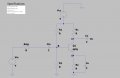First, I'm going to replace the voltage divider with a Thevenin equivalent to make things easier.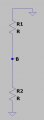$R_{th} = R1//R2 = \frac{R1R2}{R1+R2}$
$Vth = \frac{R2}{R1+R2}Vcc$

The equivalent becomes: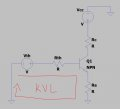Doing KVL at the loop specified in the above picture:

$$-V_{th} + I_{B}R_{th} + V_{BE} + I_{E}R_{e} = 0$$

$$I_{B}R_{th} + I_{E}R_{e} = V_{th} - V_{BE}$$

$$I_{E} = I_{C} + I_{B} = BI_{B} + I_{B} = I_{B}[B+1] <=>I_{B} = \frac{I_{E}}{B+1}$$

$$\frac{I_{E}}{B+1}R_{th} + I_{E}R_{e} = V_{th} - V_{BE}$$

$$I_{E}[\frac{R_{th}}{B+1} + R_{e}] = V_{th} - V_{BE}$$

$$I_{E} = \frac{V_{th} - V_{BE}}{\frac{R_{th}}{B+1} + R_{e}}$$

$$I_{E} = \frac{\frac{R2V_{CC}}{R1+R2} - V_{BE}}{\frac{1}{B+1}\frac{R1R2}{R1+R2} + R_{e}}$$ (1)

From equation (1), we can see how $$I_{E} \approx I_{C}$$ varies in function of B(Hfe). If we make sure
that $$R_{e} >> \frac{1}{B+1}\frac{R1R2}{R1+R2}$$ then this factor that depends on B(Hfe) can be assumed to be 0, meaning
that $$I_{E} \approx \frac{\frac{R2V_{CC}}{R1+R2} - V_{BE}}{ R_{e}}$$

Question 1) Is this the reason that people on the internet say "Choose R2 = Re/10"?

Now, let's continue with the AC Analysis: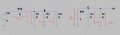KCL@B:

$$I_{in} = I_{1} + I_{2} + I_{B}$$

$$\frac{V_{in}-V_{B}}{R_{sig}} = \frac{V_{B}}{R_{1}} + \frac{V_{B}}{R_{2}} + \frac{V_{B}}{rbe}$$

$$\frac{V_{in}}{R_{sig}} = \frac{V_{B}}{R_{1}} + \frac{V_{B}}{R_{2}} + \frac{V_{B}}{rbe} + \frac{V_{B}}{R_{sig}}$$

$$\frac{V_{in}}{R_{sig}} = V_{B}[\frac{1}{R_{1}} + \frac{1}{R_{2}} + \frac{1}{rbe} + \frac{1}{R_{sig}}]$$

$$V_{in} \cdot \frac{1}{\frac{R_{sig}}{R_{1}} + \frac{R_{sig}}{R_{2}} + \frac{R_{sig}}{rbe} + 1} = V_{B}$$

$$a = \frac{R_{sig}}{R_{1}} + \frac{R_{sig}}{R_{2}} + \frac{R_{sig}}{rbe} +1$$

KCL@C:

$$BI_{B} + I_{rce} + I_{R_{C}} = 0$$

$$B\frac{V_{B}}{rbe} + \frac{V_{o}}{rce} + \frac{V_{o}}{R_{C}} = 0$$

$$B\frac{V_{in}}{a \cdot rbe} + \frac{V_{o}}{rce} + \frac{V_{o}}{R_{C}} = 0$$

$$B\frac{1}{a \cdot rbe} + \frac{V_{o}}{V_{in}}[\frac{1}{rce} + \frac{1}{R_{C}}] = 0$$

$$\frac{V_{o}}{V_{in}} = -B\frac{1}{a \cdot rbe} \cdot \frac{1}{\frac{1}{rce} + \frac{1}{R_{C}}}$$ (2)

$$rbe = (B+1)re = (B+1)\frac{B}{B+1}\frac{V_{T}}{I_{C_{dc}}} = B\frac{V_{T}}{I_{C_{dc}}} = B\frac{25mV}{I_{C_{dc}}}$$ (3)

$$rce = \frac{|A|}{I_{C_{dc}}}$$ (4)

rce
is a big resistance because $$I_{C_{dc}}$$ is in the mA range and |A|is big something like >=100
Then we can approximate (2)

$$\frac{V_{o}}{V_{in}} = -B\frac{1}{a \cdot rbe} \cdot \frac{1}{0 + \frac{1}{R_{C}}} = -B\frac{1}{a \cdot rbe} \cdot R_{C}$$

Now if we name: $$R_{b} = a \cdot rbe = \frac{rbe \cdot R_{sig}}{R_{1}} + \frac{rbe \cdot R_{sig}}{R_{2}} + 1 +rbe$$

and now (2) becomes the famous equation: $$\frac{V_{o}}{V_{in}} = -B\frac{1}{R_{b}} \cdot R_{C}$$

Also with more approximations and according to the internet, we can find out that $$R_{b} \approx rbe$$ and that makes (2) $$\frac{V_{o}}{V_{in}} = -B\frac{1}{rbe} \cdot R_{C}$$ (Which is what I see on the internet). rbe is proportional to B so it will cancel out and the gain will be independent of it.

Also at low frequencies, Re can be taken into account, and I saw on the internet that $$\frac{V_{o}}{V_{in}} = -\frac{1}{R_{e}} \cdot R_{C}$$

Calculating Zin at terminals x,y: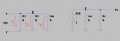KCL@B:

$$I_{1} + I_{2} + I_{B} = 0$$

$$\frac{V_{th}}{R_{1}} + \frac{V_{th}}{R_{2}} + \frac{V_{th}}{rbe} = 0 <=>V_{th} = 0$$

Question 2 (IMPORTANT QUESTION):
I've never seen this before in theory so I searched on the internet. It means that The Thevenin equivalent is independent of the input source. But how do I calculate the Zin now since I can't do $Z_{th} = \frac{V_{th}}{I_{N}} = \frac{0}{I_{N}} = 0$

I assume that you can find Zin by doing R1//R2//Rbe, but I'm not sure why, since we have a circuit with a dependent current source, and theory tells us that we can't use this technique to calculate Rth. But anyway:

$$Z_{in} = \frac{R1R2}{R1+R2} // rbe = \frac{\frac{R1R2}{R1+R2} \cdot rbe}{\frac{R1R2}{R1+R2} + rbe}$$

But can someone explain to me why is this correct when we have a dependent source in the circuit?

And since $rbe >> \frac{R1R2}{R1+R2}$

$$Z_{in} = \frac{R1R2}{R1+R2} // rbe = \frac{\frac{R1R2}{R1+R2} \cdot rbe}{\frac{R1R2}{R1+R2} + rbe \approx rbe} => Z_{in} \approx \frac{R1R2}{R1+R2}$$ (5) (What you find on the internet)

Calculating Zout at terminals z,t:

For the same reasons, we can't calculate Zout by calculating $$I_{N}$$ and $V_{th}$ , so I will assume that Zout = rce//Rc:

$$Zout = \frac{rce \cdot R_{C}}{rce + R_{C}}$$

and since rce >> Rc, then $$Zout = \frac{rce \cdot R_{C}}{rce + R_{C} \approx rce} => Z_{out} \approx R_{C}$$ (6) (Again what you see on the internet)

Phew... That was a lot of math.

Designing the actual Amp:
I will use equations (5) (6) and for the gain, I will use $$A_{v} = -\frac{R_{C}}{R_{e}}$$ which I want you to tell me why.

Specs:
Zin = 10kΩ
Zout = 2kΩ
Av = -10
frequency response 20Hz - 20KHz
Vcc = 9V

Calculating the parameters of the Amp:
Zout = Rc = 2kΩ

$$R_{e} = -\frac{Z_{C}}{A_{v}} = -\frac{2kΩ}{-10} = 200Ω$$

$$R_{2} = \frac{R_{e}}{10} = \frac{200Ω}{10} = 20Ω$$ (This feels wrong. Please tell me why).

At saturation: $$I_{C_{dc}} = \frac{V_{CC}}{R_{C}+R_{e}} = \frac{9}{2000 + 200} = 4.1mA$$

Q point: (4.1mA / 2 , 9V / 2) = (2.05mA , 4.5V)

$$V_{C} = V_{CC} - I_{C}R_{C} = 9 - 2.05 \cdot 10^{-3} \cdot 2000 = 4.9V$$

$$V_{E} = V_{C} - V_{CE} = 4.9 - 4.5 = 0.4V = 2.05 \cdot 10^{-3} \cdot 200$$

$$V_{B} \approx V_{BE} + V_{E} = 0.7 + 0.4 \approx 1.1V$$

$$I_{1} \approx I_{2}$$ because $$I_{B} \approx 0$$ since I used $R_{2} \approx \frac{R_{e}}{10}$

$$I_{1} = \frac{V_{B}}{R2} = \frac{1.1V}{20Ω} = 55mA$$

$$R_{1} = \frac{V_{CC} - V_{B}}{55 \cdot 10^{-3}} = \frac{9 - 1.1}{55 \cdot 10^{-3}} = 143.64Ω$$

$$C_{in} = \frac{1}{2 \cdot π \cdot 20 \cdot Z_{in}} = \frac{1}{2 \cdot π \cdot 20 \cdot \frac{R1R2}{R1+R2}} = \frac{1}{2 \cdot π \cdot 20 \cdot \frac{143.64 \cdot 20}{143.64+20}} = 4.53 \cdot 10^{-4}F$$

$$C_{out} = \frac{1}{2 \cdot π \cdot 20 \cdot Z_{out}} = \frac{1}{2 \cdot π \cdot 20 \cdot R_{C}} = \frac{1}{2 \cdot π \cdot 20 \cdot 2000} = 4 \cdot 10^{-6}F$$

$$C_{e} = \frac{1}{2 \cdot π \cdot 20 \cdot R_{e}} = \frac{1}{2 \cdot π \cdot 20 \cdot 200} = 4 \cdot 10^{-5}F$$

I probably got it wrong, and I think the mistake is in the Av calculating Re.

The LTspice simulations seem to agree with my calculations, though I get a weird gain in the range of Mega!!!!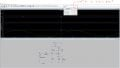Last edited:

#### dl324

Joined Mar 30, 2015
15,453
TLDR.

If you're still planning to drive a 4 ohm speaker, results from a common emitter amplifier will continue to be unsatisfactory.

•babaliaris

#### MrChips

Joined Oct 2, 2009
27,681
It does not meet the input impedance requirement.
Where did you get R2 = Re/10 ?

•babaliaris

#### babaliaris

Joined Nov 19, 2019
158
It does not meet the input impedance requirement.
Oh yes, I forgot about that.

Question a) So basically I will do $$Z{in} = \frac{R1R2}{R1+R2}$$ and since I choose R2 in an earlier stage, I can calculate R1 right?

Where did you get R2 = Re/10 ?
Is it $$R_{2} = 10\cdot R_{e}$$ ? If not then how do I find R2?

From this equation: $$I_{E} = \frac{\frac{R2V_{CC}}{R1+R2} - V_{BE}}{\frac{1}{B+1}\frac{R1R2}{R1+R2} + R_{e}}$$

$R_{e} >> \frac{1}{B+1}\frac{R1R2}{R1+R2}$

So that IE can be assumed independent from Beta (Hfe).

Question b: According to this, how do I choose the R2 resistor?

#### Audioguru again

Joined Oct 21, 2019
5,416
The resistances of R1 and R2 are way too low!
Cin and Ce have no capacitance (just numbers). 0.000453 for Cin might be 453uF?

Why is your input level only 10 MiCRO-volts peak which is almost nothing?
Then your collector level is only 200MiCRO-volts peak which is a voltage gain of 20 times.

•babaliaris

#### babaliaris

Joined Nov 19, 2019
158
The resistances of R1 and R2 are way too low!
Cin and Ce have no capacitance (just numbers). 0.000453 for Cin might be 453uF?

Why is your input level only 10 MiCRO-volts peak which is almost nothing?
Then your collector level is only 200MiCRO-volts peak which is a voltage gain of 20 times.
Can you give me an example of a "good" testing input amplitude?

Also, can you tell me how to choose R2?

Then I will try to figure out why we choose it this way.

Thank you

#### Jony130

Joined Feb 17, 2009
5,435
Question 1) Is this the reason that people on the internet say "Choose R2 = Re/10"?
In short, we want to have a "stiff" voltage divider. This means that the base current only slightly affects the output voltage of the voltage divider. And we can achieve this if we pick the voltage divider current much larger than the maximum base current. I_divider = 5...50 *Ib
The typical value for a divider current is 10*Ib (voltage divider current is ten times larger than the base current) thus:
R2 = Vb/(10*Ib)
R1 = (Vcc - Vb)/(11*Ib)

Which is almost equivalent to this rule of thumb R2 = 10*Re.

I've never seen this before in theory so I searched on the internet. It means that The Thevenin equivalent is independent of the input source. But how do I calculate the Zin now since I can't do
This is strange but ok. Zin is the "impedance seen" by a signal source, thus Zin =Vin/Iin = R1||R2||rbe

I will use equations (5) (6) and for the gain, I will use Av=−Rc/Re which I want you to tell me why.
Are you sure? Notice that you have a CE capacitor. Try to repeat the small signal analysis and find Av = Vout/Vin

Vout = -Ic*Rc||rce = β*Ib*Rc||rce
and Vin = Ib*rbe (I ignore Rsig)

Thus Av = Vout/Vin = - (β*Ib*Rc||rce )/(Ib*rbe) ≈ - (βRc)/rbe

If you remove the CE capacitor from the emitter. Then the voltage gain will be equal to:

$$\Large A_V \approx - \frac{R_C||r_{ce}}{R_E + \frac{r_{be}}{\beta +1}} \times \frac{\beta}{\beta +1} \approx \frac{R_C}{R_E + r_e}$$

Where re = Vt/Ie = 26mV/Ie

And Zin = R1||R2||(rbe + (β+1)Re) Without CE capacitor

Specs:
Zin = 10kΩ
Zout = 2kΩ
Av = -10
frequency response 20Hz - 20KHz
Vcc = 9V
Since you want to have a low gain value. You do not need a CE capacitor in your circuit.

Ic = 4V/2kΩ = 2mA
Thus re = 26mV/2mA = 13Ω
Re+re = 2kΩ/10V = 200Ω
Re = 200Ω -13Ω = 180Ω

Now the voltage divider, VE = Ic*Re = 0.36V a bit low value compared to Vbe, normally we pick Ve > Vbe. But ok. this is not a real design ---> Vb = Ve + 0.65V = 1V
Ib_max = Ic/β_min = 2mA/100 = 20μA
. But you want to have Zin = 10kΩ. We have (rbe + (β+1)Re) ≈ 20kΩ. So to get, Zin = 10kΩ the R1||R2 needs to be equal to 20kΩ.
But at the same time, we want Vb to be equal to 1V.
Vb = Vcc * R2/(R1 + R2) = 9V * R2/(R1 + R2) (1)
R1||R2 = (R1 * R2)/(R1 + R2) = 20kΩ (2)

So we could solve thous simultaneous equations to get R1 and R2 values.
R2 = 22.5kΩ ; R1 = 180kΩ
Or we could pic R1 = 20kΩ and calculated R2
I_div = 1V/20kΩ = 50μA
R2 = (Vcc - Vb)/(I_div + Ib) = (9V - 1V)/(50μA + 20μA) ≈ 110kΩ

The end of the first iteration step. See the attached file.

#### Attachments

• 1.6 KB Views: 2
Last edited:

#### Audioguru again

Joined Oct 21, 2019
5,416
Most little transistors have a minimum hFE current gain of 100. Then R1 can be about (20 times Re=) 33k and R2 can be 4.7k (24 times Re).

Your collector idle DC voltage should be half the 9V supply at 4.5V and its current is 4.5V/2k= 2.25mA.
The current in R2 should be 1/10th of the minimum hFE which would be 0.225mA and has about 1.1V across it so R2 should be 1.1V/0.225mA= 4888 ohms, use 4.7k ohms. Use the same ratio as you have (143/20) then R1 should be 33k ohms.
Cin should be about 2.2uF for good output at 20Hz.

I forgot about making the input Zin 10k ohms (it is lower).